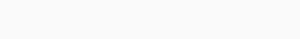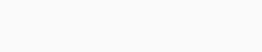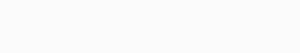#Quants Questions : Averages Set 3

Hello Aspirants. Welcome to Online Quantitative Aptitude Section with explanation in AffairsCloud.com. Here we are creating question sample in Averages, which is common for all the IBPS,SBI exam and other competitive exams. We have included Some questions that are repeatedly asked in bank exams !!!

1. The average of four consecutive ODD number is 28.Find the largest number.
A)25
B)31
C)13
D)27
Explanation :2. Find the average of first 60 natural numbers
A)30.5
B)31
C)31.5
D)32
Explanation :3. Find  the  average  of  13+26+39+……..+260
A)136.5
B)136
C)137
D)135
Explanation :4. The average of five number is 42 ,if one number is excluded  the average become 35.The excluded number is
A)7
B)40
C)70
D)20
Explanation :5. The average age of  30 students in a class is 20 years.The average age of 25 students is 15.What is the average age of remaining students
A)42
B)54
C)34
D)45
Explanation :6. The average of  nine number is x and the average of three of these is y,if the average of the remaining three is z,then
A)3x=y+z
B)2x=y+z
C)x=3y+3z
D)None of these
Explanation :7. If a,b,c,d,e,f,g  are seven consecutive odd number,their average is
A)(a+6)
B)(abcdefg/7)
C)(a+b+c+d+e+f+g)/7
D)None of these
Explanation :8. The mean of 1,8,27,64,125………1728
A)650
B)560
C)600
D)605
Explanation :9. 4 years ago ,the average age of a family of 6member  was 20 years.A baby having been born,the average age of  the  family is same today.The present age of the baby
A)1
B)3
C)2
D)4
Explanation :10. Average of ten positive numbers  is x.If each number is increased by 12%  then x is incresed by
A)5%
B)12%
C)10%
D)25%